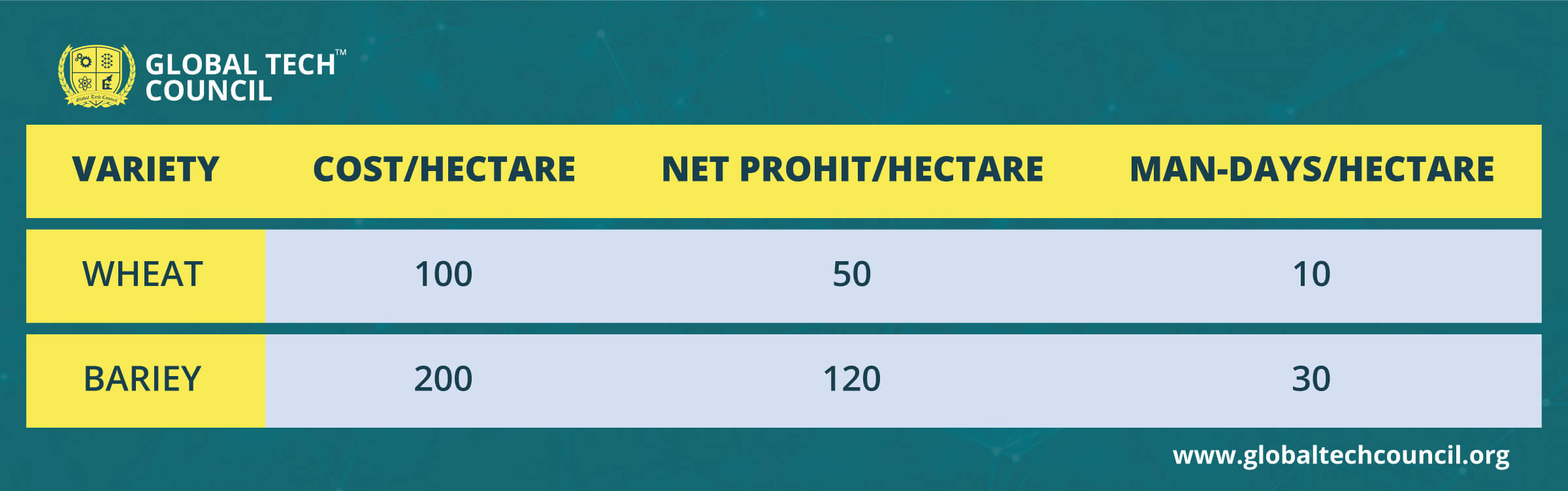# A Quick Guide to Linear Programming For Data Science

As a data science expert, one is required to make informed and data-driven decisions from time to time. Even in daily life, one deals with situations involving optimization, especially when there are finite resources and, like packing a suitcase for a vacation or sharing a pack of cookies between siblings. As the complexity of such a problem increases, the concept of Linear programming comes in handy for data science developers.

Linear Programming (LP) is the most effective way to go about optimization and has found its grounding in many applications and has become a core topic in data science training.

## Learning of Blog

• What is Linear Programming?
• Formulation of Linear Programming Problem
• How to solve a Linear Programming Problem
• Graphical Method
• Simplex Method
• Northwest and Least Cost Method
• Solving using R
• Solving using OpenSolver
•  Example of LPP
• Conclusion

As a data scientist, one comes across Linear programming problems, which are also a part of high school and engineering mathematics. If you cannot recall, no worries- this article has got you covered!

## What is Linear Programming?

Linear Programming is a scientific technique used in Operations Research to find optimum solutions to a given business problem. It helps find the best outcome by representing complex relationships through linear functions. LP can be used to solve personal and professional problems by taking limitations or constraints into account. Linear programming is a part of the predictive analysis and covered in every handbook on data science for beginners.

It is essential to understand the basics of Linear Programming before we can start using it in Data Science.

## Formulation of a Linear Programming Problem

For the formulation of a linear program, it is essential to keep the following concepts in mind:

• The relationships that need to be simplified must be linear.

• The values, either in number or in properties must be subject to a constraint.

• The goal is to find a set of values that maximize or minimize the objective function while satisfying all the constraints.
• The value of decision variables should be non-negative.

All these terms would become more apparent when we discuss some examples in the upcoming sections.

## Solving a Linear Programming Problem

There are several methods to solve a Linear Problem. Here, we shed light on some commonly used techniques:

• ### Graphical Method

This method solves a linear program in two variables. It works best when there are only two decision variables. It involves the formulation of a set of linear inequalities subject to the conditions. This step is followed by plotting on the X-Y plane. The area of intersection formed by plotting all linear equations gives the feasible region containing the solution. The feasible region indicates the values a model can have and provides the optimal solution.

• ### Simplex Method

A very powerful and popular mechanism, the simplex method gives the optimal solution by an iterative procedure. In this method, the value of basic variables keeps getting modified until we get a maximum or minimum value for the objective function.

• ### Northwest Corner and Least Cost Method

These are particular types of methods used for transportation problems in linear programming to decide the best way to transport commodities. These are generally used for supply-demand problems and depend on the quantity of demand and supply at each source and the unit price of transportation. The assumption is that there is only one commodity, and demand comes from various sources, which are also equal to total supply. The aim is to minimize the cost of transportation. Least Corner is more efficient than the Northwest Corner Method.

• ### Solving using R

R is an open-source statistical tool heavily used by data scientists for necessary tasks. It is very easy to perform optimization on R by using a few code lines because of its package ‘lpSolve.’

• ### Solving using OpenSolver

For a problem that contains up to 100 variables, OpenSolver is a blessing. It is a linear optimizer for Microsoft Excel and works as an advanced version of the built-in Solver.

Gurobi, R, CPLEX, Open Solver, MATLAB, GAM S, SAS, Mathematica, SciPy, PulP, Pyomo are some of the available open-source tools that serve the purpose.

Further, let us solve an example using the Graphical Method as it is easy to comprehend and found in the best data science programs online.

### Example of LPP

Problem Statement: A farm owner has 110 hectares of land. He grows Wheat and Barley on it. He wants to sow in such a manner as to maximize his profits according to:He has a budget of 10,000 units and availability of 1,200 man-days.

Let us go solve this step by step:

1.     Decision Variables- X (area for growing wheat) and Y (area for growing barley)
2.     Objective Function: Z= 50X +120Y
3.     Constraints: 100X +200Y <=10000, 10X + 30Y <=1200 and X+Y <=110
4.     Non negativity: X, Y >=0
5.     Plotting simplified equations: X +2Y <=100, X + 3Y <=120, X +Y<=110
6. The solution is achieved at the point of intersection given the budget & man-days constraints are active. This means the point of intersection of equations X + 2Y ≤ 100 and X + 3Y ≤ 120 gives optimal solution P(60,20)
7.   Max Z = 50 * (60) + 120 * (20) = 5400 units

## Conclusion

As it is evident, LPP can be used to investigate complex situations and get seamless results for your business problems. Understanding the optimization theory is essential for Data Science, and if you wish to try it out and practice hands-on – Data Science Certification is for you.